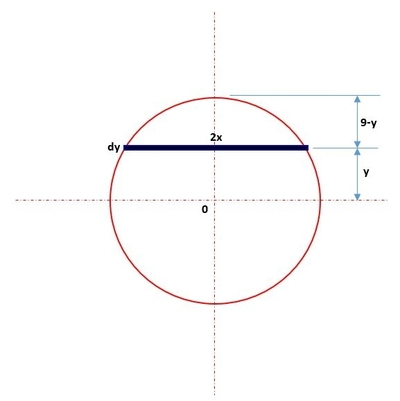# A spherical tank of radius 9 mts is filled with water. Find the work performed against gravity in...

## Question:

A spherical tank of radius {eq}9{/eq} mts is filled with water. Find the work performed against gravity in pumping out the water through a small hole at the top

## Work Done:

Recall that the formula for work is {eq}W=\int F\cdot d {/eq} where {eq}F {/eq} is the force and {eq}d {/eq} is the distance. However, force is also equal to {eq}F=\rho gV {/eq} the product of the density {eq}\rho {/eq} , acceleration due to gravity {eq}g {/eq} and the volume.

Become a Study.com member to unlock this answer! Create your account

Below is the graph,From the figure,

{eq}W=\int_{-9}^{9}\rho g\left ( \pi x^{2}dy \right )\cdot \left ( 9-y \right ) {/eq}

{eq}\display...Work as an Integral

from

Chapter 7 / Lesson 9
18K

After watching this video, you will be able to solve calculus problems involving work and explain how that relates to the area under a force-displacement graph. A short quiz will follow.